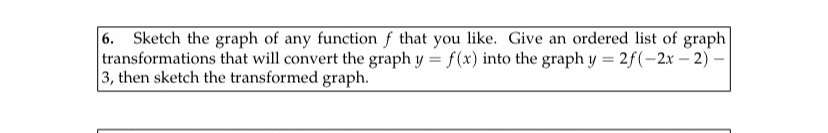### ¿Todavía tienes preguntas de matemáticas?

Pregunte a nuestros tutores expertos
Algebra
Pregunta6. Sketch the graph of any function $$f$$ that you like. Give an ordered list of graph transformations that will convert the graph $$y = f ( x )$$ into the graph $$y = 2 f ( - 2 x - 2 ) -$$ $$3$$ , then sketch the transformed graph.

$$f ( x ) = 5 x$$
$$2 ( - 10 x - 2 ) - 3$$